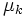# Friction

## Definition

### Intensional definition

Friction (sometimes called sliding friction to distinguish from rolling friction) is the component of contact force between two bodies with a surface of contact acting in a direction along (or parallel to) the surface of contact, or the tangent plane through the point of contact.

### Tendency-based definition

Friction refers to a force exerted by two bodies on each other, through a surface of contact, that tends to oppose slipping of the two surfaces of contact against each other. Note that static friction opposes the tendency indicated by the direction of net external force along the plane of contact, while kinetic friction opposes the direction of actual motion.

## Related forces

### Types of friction

• Static friction: This is friction exerted when the surfaces of contact are not slipping relative to each other. This occurs, for instance, both when the surfaces are stationary, and in the case of rolling. Static friction acts to precisely cancel the net external force along the plane of contact. The maximum possible value of static friction is$\mu_sN$ where$N$ is the normal force and$\mu_s$ is the limiting coefficient of static friction for the two surfaces.
• Kinetic friction or dynamic friction: This is friction exerted when the surfaces are slipping relative to each other. Kinetic friction acts in the direction opposite to the direction of slipping, and for small relative velocities, its magnitude is$\mu_kN$, where$N$ is the normal force and$\mu_k$ is the coefficient of kinetic friction.

### Forces between rigid bodies

• Rolling friction: This is not really friction, but rather, a normal force that effectively plays the role of friction due to local deformations of the surface.
• Normal force: The component of contact force in the direction perpendicular to the plane of contact.